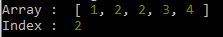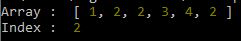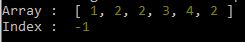Open in App
Not now

# Lodash _.lastIndexOf() Method

• Last Updated : 03 Aug, 2020

The lodash _.indexOf() method is used to get the index of the first occurrence of the particular element in the array from the last element. If fromIndex is not present in the array negative one is given as the output and no error is displayed. This method is like lodash  _.indexOf except that it iterates over elements of an array from right to left.

Syntax:

```_.lastIndexOf(array, value, [fromIndex=array.length-1])
```

Note: If the value is not found in the array -1 is returned.

Parameters: This method accepts three parameters as mention above and described below.

• array: It is the array in which value is to be found.
• value: It is the value to be looked in the array.
• fromIndex: It is the index after which we have to look for the value.

Return Value: It returns the index of the value in the array. If the value is not found, the array returned -1.

Example 1:

## Javascript

 `// Requiring the lodash library ``const _= require(``"lodash"``); ``   ` `// Original array ``let array = [1, 2, 2, 3, 4] ``   ` `// Printing original array  ``console.log(``"Array : "``,array) ``   ` `// Looking for value 3 from Last index   ``let index = _.lastIndexOf(array,2) ``   ` `// Printing the Index of the value  ``console.log(``"Index : "``,index)`

Output:Example 2: Looking for a value from a particular index.

## Javascript

 `// Requiring the lodash library ``const _= require(``"lodash"``); ``   ` `// Original array ``let array = [1, 2, 2, 3, 4, 2] ``   ` `// Printing original array  ``console.log(``"Array : "``,array) ``   ` `// Looking for value 3 from Last index   ``let index = _.lastIndexOf(array,2,2) ``   ` `// Printing the Index of the value  ``console.log(``"Index : "``,index)`

Output:Example 3: Looking for a value that is not present.

## Javascript

 `// Requiring the lodash library ``const _= require(``"lodash"``); ``   ` `// Original array ``let array = [1, 2, 2, 3, 4, 2] ``   ` `// Printing original array  ``console.log(``"Array : "``,array) ``   ` `// Looking for value 3 from Last index   ``let index = _.lastIndexOf(array,4,2) ``   ` `// Printing the Index of the value  ``console.log(``"Index : "``,index)`

Output:My Personal Notes arrow_drop_up